# PSAT Math : How to find absolute value

## Example Questions

### Example Question #1 : Number Lines And Absolute Value

If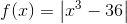, what is the value of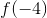?

Possible Answers: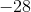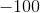Correct answer:Explanation:

Substitute – 4 in for x. Remember that when a negative number is raised to the third power, it is negative. -  = – 64. – 64 – 36 = – 100. Since you are asked to take the absolute value of – 100 the final value of f(-4) = 100. The absolute value of any number is positive.

### Example Question #1 : How To Find Absolute Value

For which of the following functions below does f(x) = |f(x)| for every value of x within its domain?

Possible Answers:

f(x) = x2 – 2x

f(x) = 2x + 3

f(x) = x2 – 9

f(x) = x4 + (1 – x)2

f(x) = x4 + x

Correct answer:

f(x) = x4 + (1 – x)2

Explanation:

When we take the absolute value of a function, any negative values get changed into positive values. Essentially, |f(x)| will take all of the negative values of f(x) and reflect them across the x-axis. However, any values of f(x) that are positive or equal to zero will not be changed, because the absolute value of a positive number (or zero) is still the same number.

If we can show that f(x) has negative values, then |f(x)| will be different from f(x) at some points, because its negative values will be changed to positive values. In other words, our answer will consist of the function that never has negative values.

Let's look at f(x) = 2x + 3. Obviously, this equation of a line will have negative values. For example, where x = –4, f(–4) = 2(–4) + 3 = –5, which is negative. Thus, f(x) has negative values, and if we were to graph |f(x)|, the result would be different from f(x). Therefore, f(x) = 2x + 3 isn't the correct answer.

Next, let's look at f(x) = x2 – 9. If we let x = 1, then f(1) = 1 – 9 = –8, which is negative. Thus |f(x)| will not be the same as f(x), and we can eliminate this choice as well.

Now, let's examine f(x) = x2 – 2x. We know that x2 by itself can never be negative. However, if x2 is really small, then adding –2x could make it negative. Therefore, let's evaluate f(x) when x is a fractional value such as 1/2. f(1/2) = 1/4 – 1 = –3/4, which is negative. Thus, there are some values on f(x) that are negative, so we can eliminate this function.

Next, let's examine f(x) = x4 + x. In general, any number taken to an even-numbered power must always be non-negative. Therefore, x4 cannot be negative, because if we multiplied a negative number by itself four times, the result would be positive. However, the x term could be negative. If we let x be a small negative fraction, then x4 would be close to zero, and we would be left with x, which is negative. For example, let's find f(x) when x = –1/2. f(–1/2) = (–1/2)+ (–1/2) = (1/16) – (1/2) = –7/16, which is negative. Thus, |f(x)| is not always the same as f(x).

By process of elimination, the answer is f(x) = x4 + (1 – x)2 . This makes sense because x4 can't be negative, and because (1 – x)can't be negative. No matter what we subtract from one, when we square the final result, we can't get a negative number. And if we add x4 and (1 – x)2, the result will also be non-negative, because adding two non-negative numbers always produces a non-negative result. Therefore, f(x) = x4 + (1 – x)will not have any negative values, and |f(x)| will be the same as f(x) for all values of x

The answer is f(x) = x4 + (1 – x)2 .

### Example Question #2 : How To Find Absolute Value

Letandboth be negative numbers such that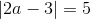and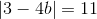. What is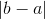?

Possible Answers:Correct answer:Explanation:

We need to solve the two equations |2a – 3| = 5 and |3 – 4b| = 11, in order to determine the possible values of a and b. When solving equations involving absolute values, we must remember to consider both the positive and negative cases. For example, if |x| = 4, then x can be either 4 or –4.

Let's look at |2a – 3| = 5. The two equations we need to solve are 2a – 3 = 5 and 2a – 3 = –5.

2a – 3 = 5 or 2a – 3 = –5

Add 3 to both sides.

2a = 8 or 2a = –2

Divide by 2.

a = 4 or a = –1

Therefore, the two possible values for a are 4 and –1. However, the problem states that both a and b are negative. Thus, a must equal –1.

Let's now find the values of b.

3 – 4b = 11 or 3 – 4b = –11

Subtract 3 from both sides.

–4b = 8 or –4b = –14

Divide by –4.

b = –2 or b = 7/2

Since b must also be negative, b must equal –2.

We have determined that a is –1 and b is –2. The original question asks us to find |b – a|.

|b – a| = |–2 – (–1)| = | –2 + 1 | = |–1| = 1.

The answer is 1.

### Example Question #1 : How To Find Absolute Value

Evaluate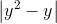if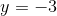.

Possible Answers:Correct answer:Explanation:

Remember that the absolute value of a number is the positive value of that number. It is important to know what absolute value represents: the distance of any given number from the number 0. For that reason, absolute value cannot be negative, and we can eliminateandfrom our answer choices

To solve, substituteinto the equation, taking extra care to correctly calculate the negative numbers: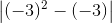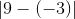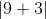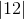The answer is 12.

### Example Question #3 : How To Find Absolute Value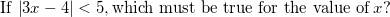Possible Answers: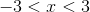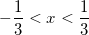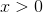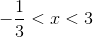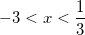Correct answer:Explanation: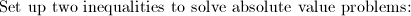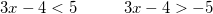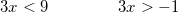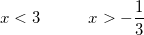### Example Question #1 : How To Find Absolute Value

How many of these statements are correct?

I)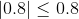II)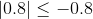III)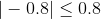IV)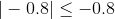Possible Answers:

Two

One

None

Three

Four

Correct answer:

Two

Explanation:

The absolute value of a nonnegative number is the number itself; the absolute value of a negative number can be obtained by removing the negative symbol. Therefore,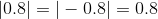.

The four statements can be rewritten as:

Both I and III)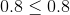- This is true.

Both II and IV)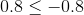- This is false.

The correct response is two.

### All PSAT Math Resources